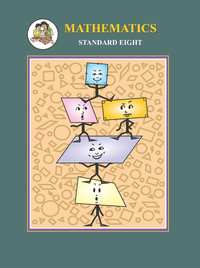Share

# Balbharati solutions for Maharashtra state board (SSC) Class 8 Mathematics chapter 3 - Indices and Cube root [Latest edition]

Textbook page

#### Chapters## Chapter 3: Indices and Cube root

Practice Set 3.1Practice Set 3.2Practice Set 3.3

### Balbharati solutions for Maharashtra state board (SSC) Class 8 Mathematics Chapter 3 Indices and Cube root Practice Set 3.1 [Page 15]

Practice Set 3.1 | Q 1.1 | Page 15

Express the following number in index form.

Fifth root of 13

Practice Set 3.1 | Q 1.2 | Page 15

Express the following number in index form.

Sixth root of 9

Practice Set 3.1 | Q 1.3 | Page 15

Express the following number in index form.

Square root of 256

Practice Set 3.1 | Q 1.4 | Page 15

Express the following number in index form.

Cube root of 17

Practice Set 3.1 | Q 1.5 | Page 15

Express the following number in index form.

Eighth root of 100

Practice Set 3.1 | Q 1.6 | Page 15

Express the following number in index form.

Seventh root of 30

Practice Set 3.1 | Q 2.1 | Page 15

Write in the form 'nth root of a' in the following number.

(81)1/4

Practice Set 3.1 | Q 2.2 | Page 15

Write in the form 'nth root of a' in the following number.

49^(1/2)

Practice Set 3.1 | Q 2.3 | Page 15

Write in the form 'nth root of a' in the following number.

(15)^(1/5)

Practice Set 3.1 | Q 2.4 | Page 15

Write in the form 'nth root of a' in the following number.

(512)^(1/9)

Practice Set 3.1 | Q 2.5 | Page 15

Write in the form 'nth root of a' in the following number.

100^(1/19)

Practice Set 3.1 | Q 2.6 | Page 15

Write in the form 'nth root of a' in the following number.

(6)^(1/7)

### Balbharati solutions for Maharashtra state board (SSC) Class 8 Mathematics Chapter 3 Indices and Cube root Practice Set 3.2 [Page 16]

Practice Set 3.2 | Q 1 | Page 16

Complete the following table.

 Sr. No. Number Power of the root Root of the power (1) (225)3/2 Cube of square root of 225 Square root of cube of 225 (2) (45)4/5 (3) (81)6/7 (4) (100)4/10 (5) (21)3/7
Practice Set 3.2 | Q 2.1 | Page 16

Write the following number in the form of rational indices.

Square root of 5th power of 121

Practice Set 3.2 | Q 2.2 | Page 16

Write the following number in the form of rational indices.

Cube of 4th root of 324

Practice Set 3.2 | Q 2.3 | Page 16

Write the following number in the form of rational indices.

5th root of square of 264

Practice Set 3.2 | Q 2.4 | Page 16

Write the following number in the form of rational indices.

Cube of cube root of 3

### Balbharati solutions for Maharashtra state board (SSC) Class 8 Mathematics Chapter 3 Indices and Cube root Practice Set 3.3 [Page 18]

Practice Set 3.3 | Q 2.1 | Page 18

Simplify:

root(3)(27/125)

Practice Set 3.3 | Q 2.2 | Page 18

Simplify:

root(3)(16/54)

Practice Set 3.3 | Q 3 | Page 18

If root(3)(729) = 9 then root(3)(0.000729) = ?

Practice Set 3.3 | Q 3.1 | Page 18

Find the cube root of the following number.

8000

Practice Set 3.3 | Q 3.2 | Page 18

Find the cube root of the following number.

729

Practice Set 3.3 | Q 3.3 | Page 18

Find the cube root of the following number.

343

Practice Set 3.3 | Q 3.4 | Page 18

Find the cube root of the following number.

-512

Practice Set 3.3 | Q 3.5 | Page 18

Find the cube root of the following number.

-2744

Practice Set 3.3 | Q 3.6 | Page 18

Find the cube root of the following number.

32768

## Chapter 3: Indices and Cube root

Practice Set 3.1Practice Set 3.2Practice Set 3.3## Balbharati solutions for Maharashtra state board (SSC) Class 8 Mathematics chapter 3 - Indices and Cube root

Balbharati solutions for Maharashtra state board (SSC) Class 8 Mathematics chapter 3 (Indices and Cube root) include all questions with solution and detail explanation. This will clear students doubts about any question and improve application skills while preparing for board exams. The detailed, step-by-step solutions will help you understand the concepts better and clear your confusions, if any. Shaalaa.com has the Maharashtra State Board Maharashtra state board (SSC) Class 8 Mathematics solutions in a manner that help students grasp basic concepts better and faster.

Further, we at Shaalaa.com provide such solutions so that students can prepare for written exams. Balbharati textbook solutions can be a core help for self-study and acts as a perfect self-help guidance for students.

Concepts covered in Maharashtra state board (SSC) Class 8 Mathematics chapter 3 Indices and Cube root are Indices and Cube Root, Meaning of Numbers with Rational Indices, Cube and Cube Root.

Using Balbharati Class 8 solutions Indices and Cube root exercise by students are an easy way to prepare for the exams, as they involve solutions arranged chapter-wise also page wise. The questions involved in Balbharati Solutions are important questions that can be asked in the final exam. Maximum students of Maharashtra State Board Class 8 prefer Balbharati Textbook Solutions to score more in exam.

Get the free view of chapter 3 Indices and Cube root Class 8 extra questions for Maharashtra state board (SSC) Class 8 Mathematics and can use Shaalaa.com to keep it handy for your exam preparation